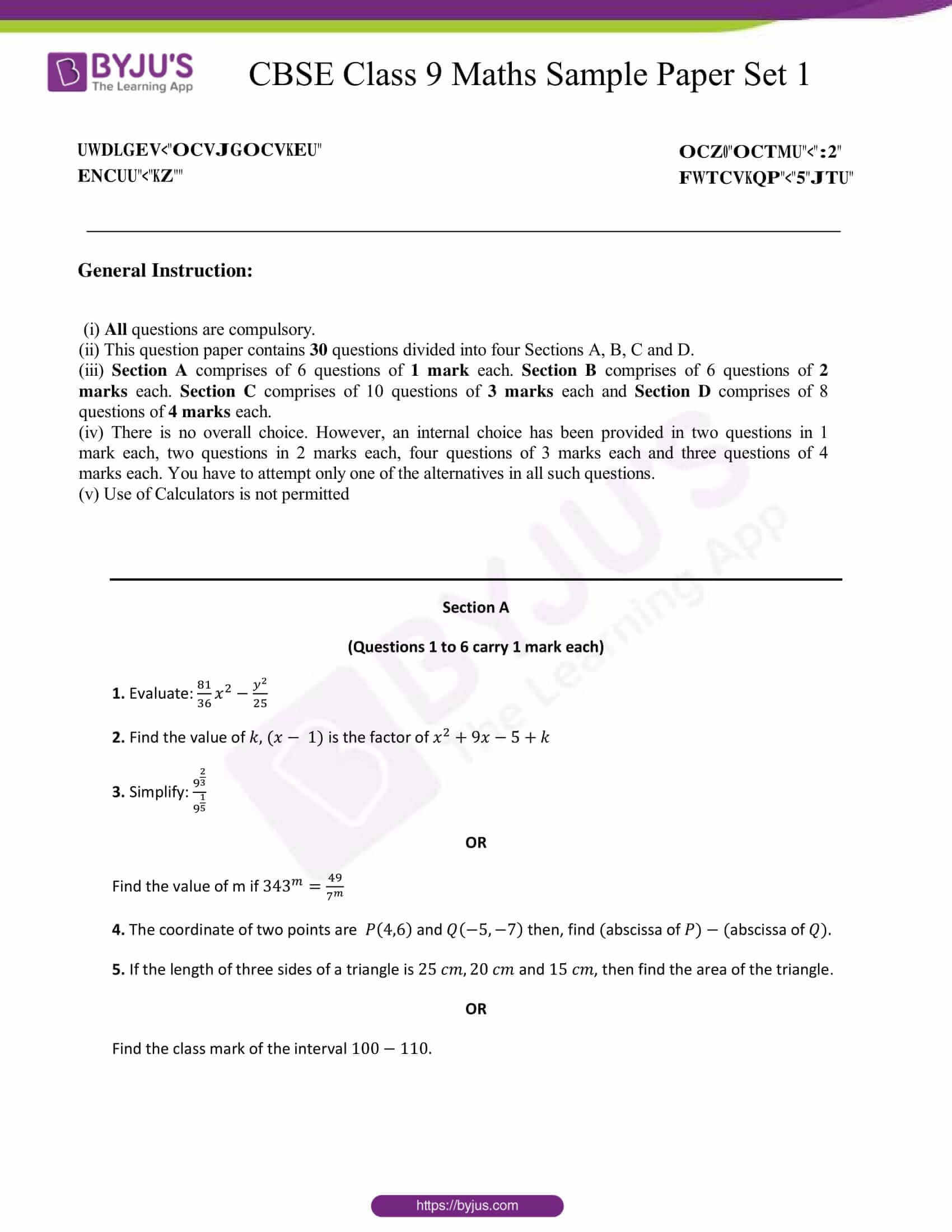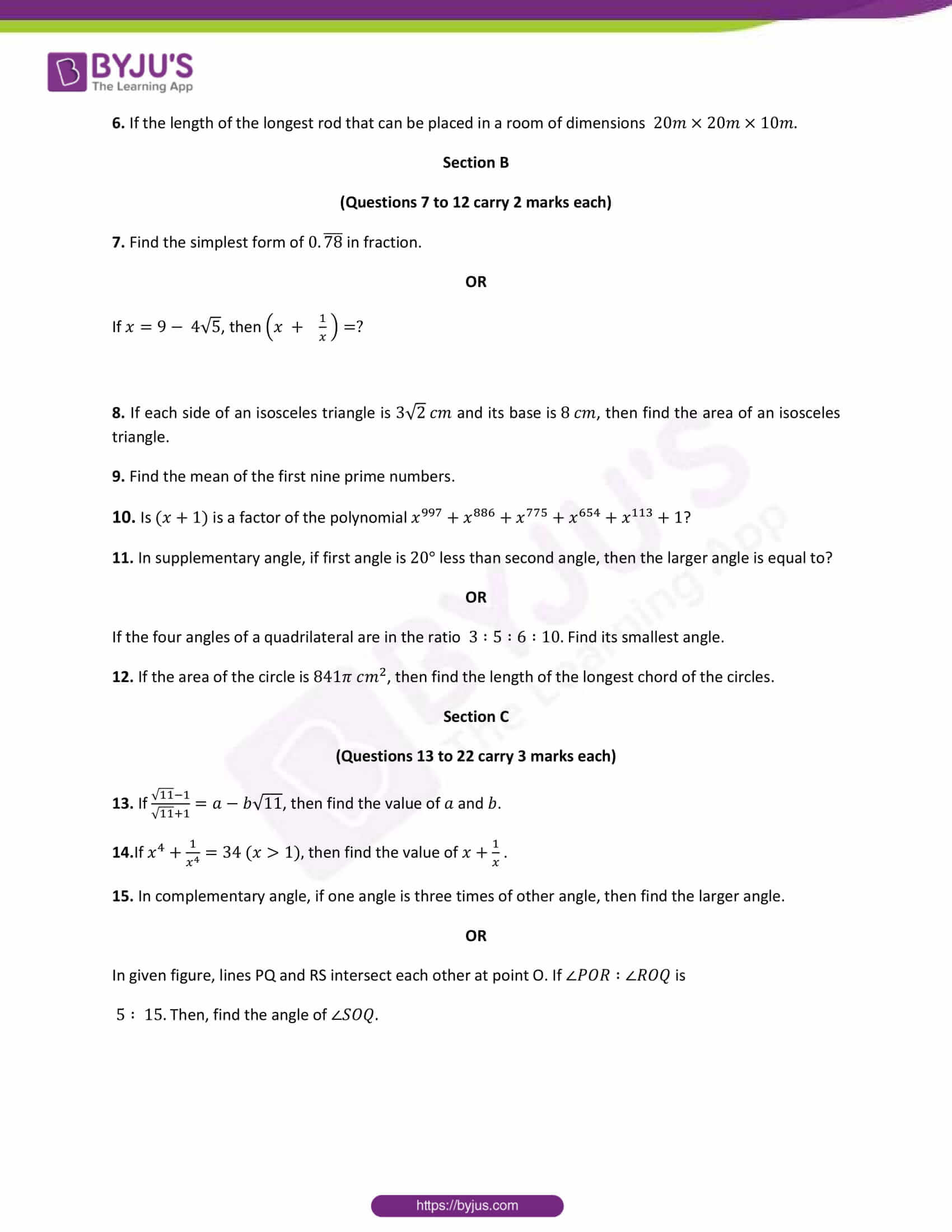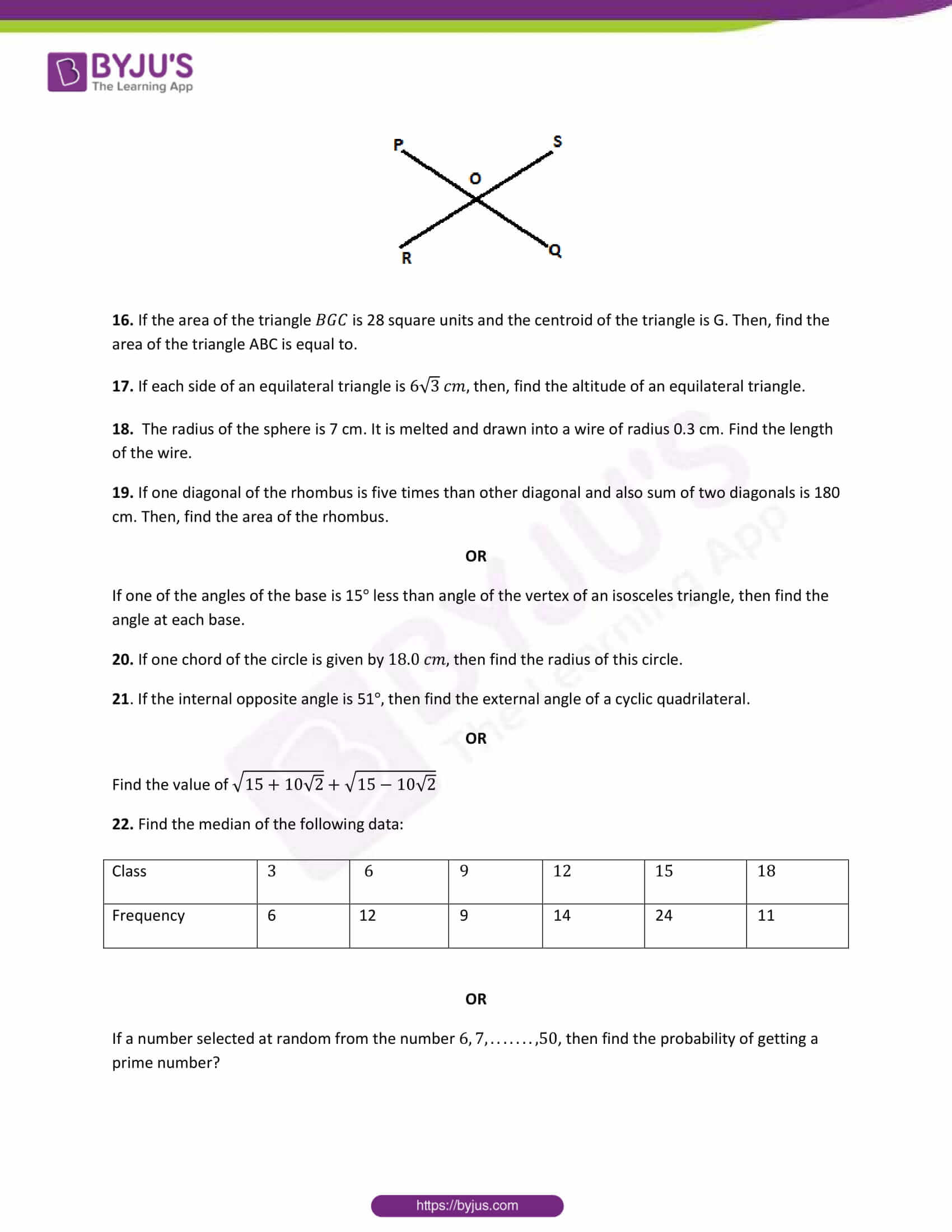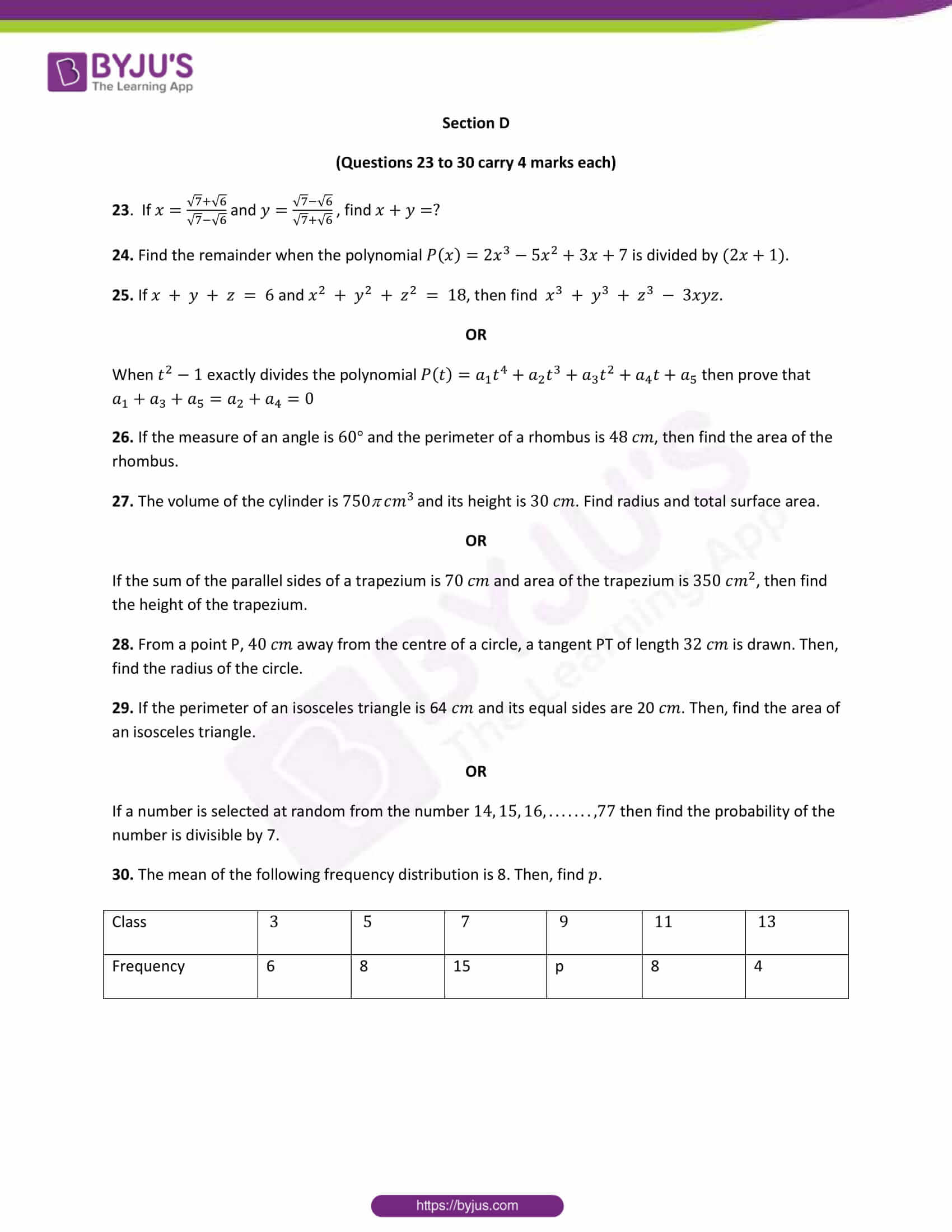# CBSE Sample Paper for Class 9 Maths Set 1

Maths is a subject that is purely based on numbers. For some students, it is an interesting subject, but some of them find it difficult. One of the best ways to overcome this Maths fear is to strengthen the basic concepts and practice questions based on them. Once students are done with solving the textbook problems, then they must solve the questions of varying difficulty level. Here we have provided the CBSE Sample Paper Class 9 Maths Set 1 which include different types of Maths problems. Students must solve this paper after completing their syllabus. It will boost their confidence level and help them to score high marks in Maths exam.### Access Other Sets of CBSE Class 9 Maths Sample Papers

Students can access the different sets of CBSE Class 9 Sample Papers for Maths from the table below:

By practising the CBSE Class 9 Maths Sample Papers, students can evaluate their current preparation level. Also, they get to know their weak and strong areas.

### Benefits of Solving the CBSE Class 9 Maths Sample Papers

Here we have listed a few benefits of solving the CBSE Sample Papers.

1. Students understand the exam pattern and marking scheme.
2. By practising the sample papers, students could able to finish the paper on time.
3. They get to know the types of questions asked in the Maths papers and their difficulty level.
4. Students can check their current exam preparation by solving the sample papers.
5. They get confidence to face the exam boldly.

We hope students find this information on “CBSE Sample Paper for Class 9 Maths Set 1” helpful in exam preparation. Keep learning and stay tuned for further updates on CBSE and other competitive exams. Download BYJU’S App and subscribe to YouTube channel to access interactive Maths and Science videos.